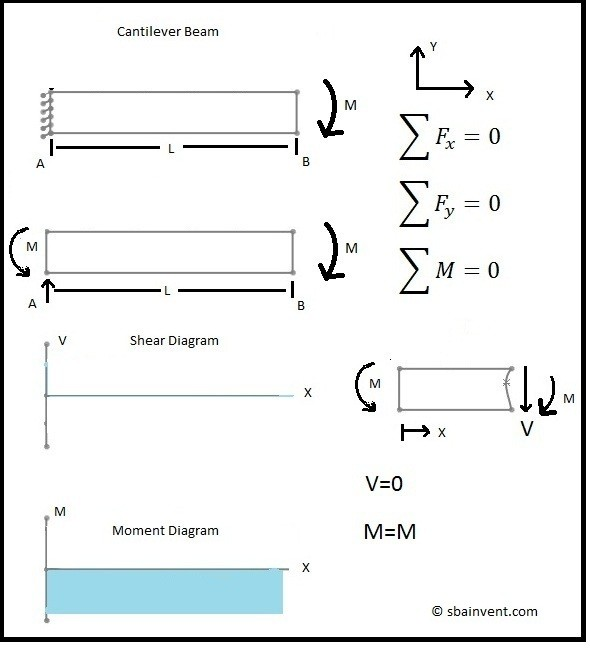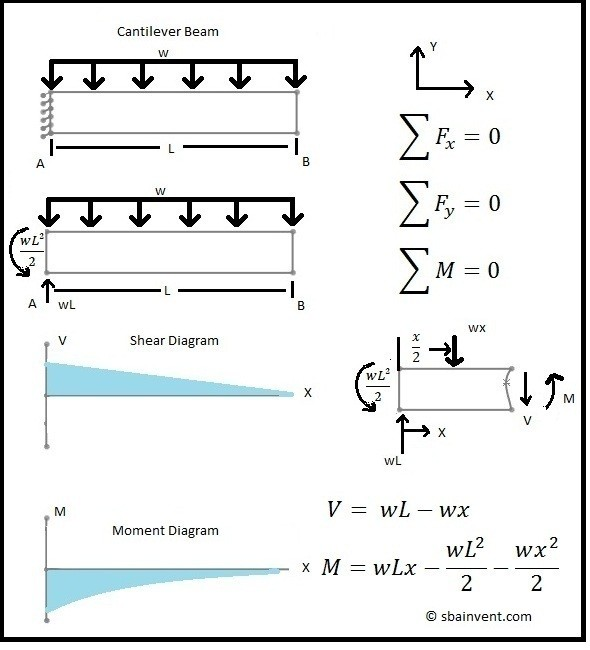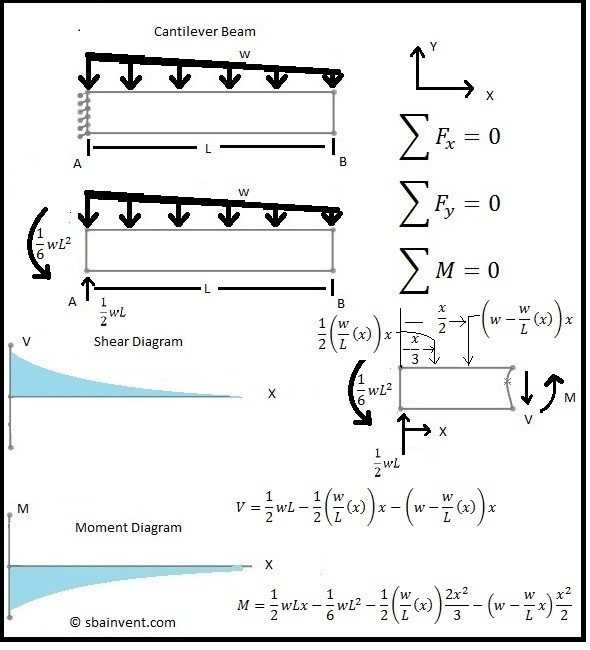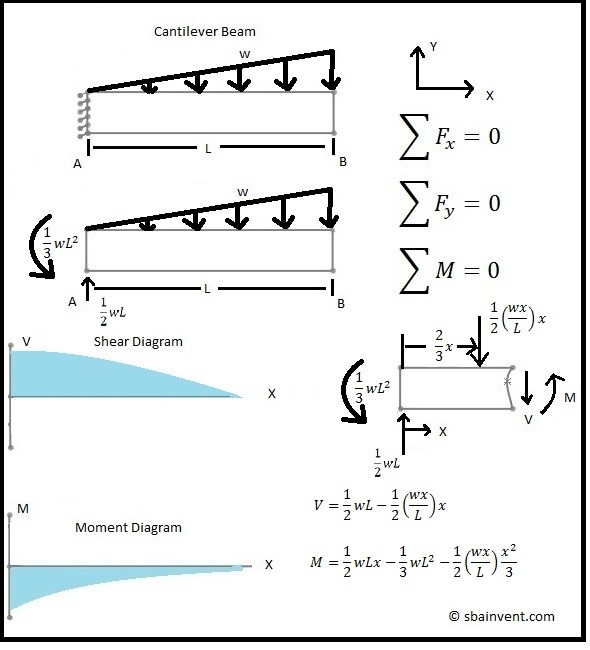# Shear and Moment Diagrams

Understanding how to solve beam problems in strength materials is very important. This is because most parts in a structure could be considered beams. In most cases these beams would be statically indeterminate. First, I will discuss beam problems that can be solved using statics.

The first part of being able to calculate a beam problem is to be able to calculate the shear and moment diagrams, and the equations that represent these diagrams. Using statics and creating free body diagrams are very useful in determining these diagrams. Below you can see 5 cases for a cantilever beam and 1 case for simply supported beam. There are an infinite number of problems that can be derived with different types of forces. These problems show the basics.

Once the shear and moment diagrams and their corresponding equations are determined. The moment and shear at any point in the beam can be determined. By having this information, the normal and shear stress at certain points of the beam can then be calculated.